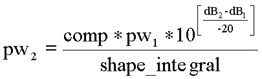Tools Home Molecular Biology NMR Data Model VENN Links
UCHC>SBF>TOOLS_HOME>NMR>PW_CALCULATOR

• Machine - The type of NMR instrument you are using.
• Reference PW - The pulse width of the hard pulse at high power.
• Reference Power - The power level used to calibrate the reference pw.
• Compression - The correction factor to compensate for the non-linearity of the linear amplifiers at high output levels normally used for hard pulses.
• Shape Factor - A correction factor to compensate for the type of shaped pulse you are using.
• Calculate - Determines whether you want to calculate pw from a given power level or whether you want to calculate the power level for a given pw.
• Input - Either the pw or power level depending on your selection for Calculate.
 Machine Varian Bruker Reference PW us Reference Power dB Compression Shape Factor Calculate Pulse Width Power Level Input

Theory
If a pulse width has been calibrated at a given power level then the pulse width at any other power level can be calculated mathematically without any need for experimentally calibrating the pulse. The opposite, that is calculating the power level for a given pulse width, is also possible. This can be very useful for pulses with long pulse widths, shaped pulses, and off resonance pulses, all of which may be very difficult to determine experimentally.
• For every 3 dB change in the power level the power produced by the amplifier will either increase or decrease two fold.
• For every 6 dB change in power the voltage applied to the probe coil is either increased or decreased two fold.
• Field strength (pulse width) is proportional to voltage (not power), therefore for every 6 dB change in power the pulse width is increased or decreased by a factor of two.
From the 6 dB relationship between pulse width and pulse power the following equation can be derived.However, this equation assumes perfect linearity of the amplifiers and assumes the shape of pw1 is the same as pw2. In practice, hard pulses are set up to have the smallest pulse width without causing arcing in the probe. At these high powers the amplifiers become non-linear. This non-linearity can be calibrated and easily compensated for in the equation by introduction of a comp value, where comp equals:Shaped pulses will need more power to achieve the same pulse width as a rectangular pulse and the amount of extra power needed is dependent upon the integral of the shape as compared to a rectangular pulse. The shape integrals can easily be determined by using the programs pulsetool and shapetool on Varian and Bruker instruments, respectively. Taking the amplifier compression and shape pulse into account we get:and rearranging to solve for pw2 we get:NOTE: The above equations assume an NMR instrument with linear amplifiers and are written for calculations for Varian instruments where power levels increase with increasing dB values. For Bruker instruments, which have increasing power levels with decreasing dB values, the sign before the 20 should be changed.